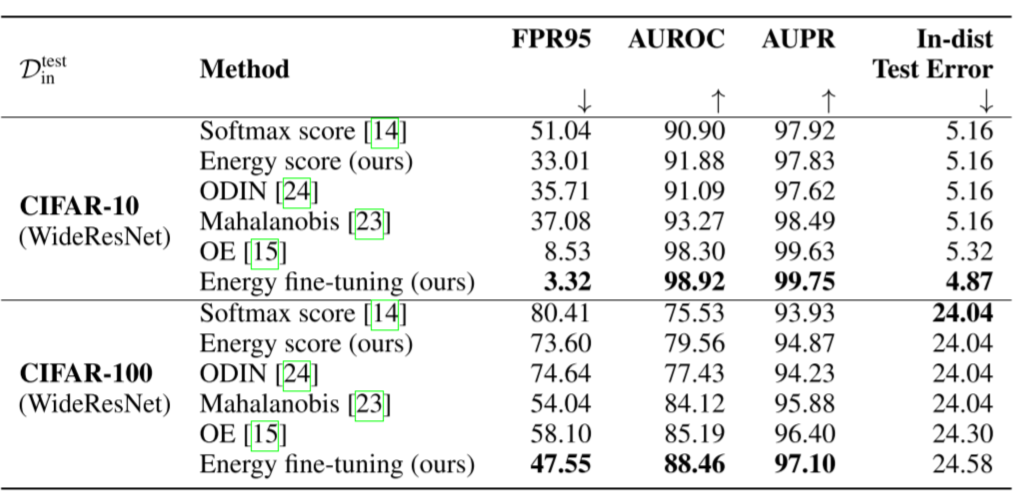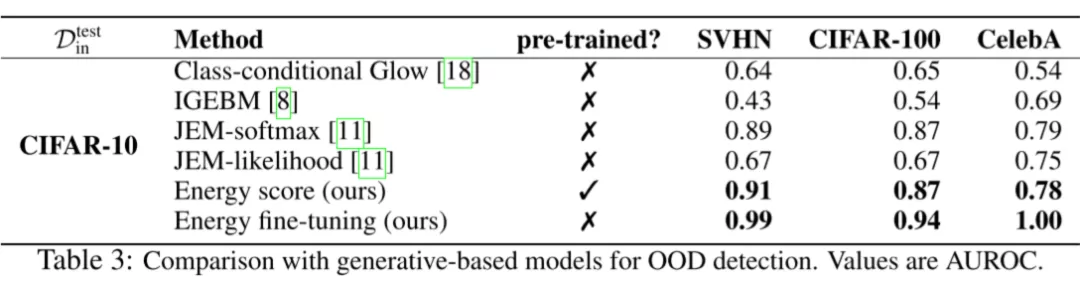# Energy-based Out-of-distribution Detection

## Introduction

OOD（Out-of-distribution）检测的传统方法之一是基于 softmax confidence。直觉上来看，对于 in distribution 的数据点，我们有高可信度给他们一个结果（就分类问题而言即将一张猫的图片分类为“猫”的概率很高），那么可信度低的就是 OOD inputs。但是因为 DNN 在样本空间的过拟合，经常会对OOD的样本（比如对抗样本）一个很高的可信度。

## Background: Energy-based Models

$$p(y \mid \mathbf{x})=\frac{e^{-E(\mathbf{x}, y) / T}}{\int_{y^{\prime}} e^{-E\left(\mathbf{x}, y^{\prime}\right) / T}}=\frac{e^{-E(\mathbf{x}, y) / T}}{e^{-E(\mathbf{x}) / T}}$$

$$E(\mathbf{x})=-T \cdot \log \int_{y^{\prime}} e^{-E\left(\mathbf{x}, y^{\prime}\right) / T}$$

$$p(y \mid \mathbf{x})=\frac{e^{f_{y}(\mathbf{x}) / T}}{\sum_{i}^{K} e^{f_{i}(\mathbf{x}) / T}}$$

$$E(x, f)=-T \log \sum_{i=1}^{K} e^{f_{i}(x) / T}$$

## Energy-based Out-of-distribution Detection

### Energy as Inference-time OOD Score

OOD是一个二分类问题，判别IND or OOD。自然想到可以利用数据的密度函数，认为低似然的例子为OOD。而利用基于能量的模型可以得到判别模型的密度函数：
$$p(\mathbf{x})=\frac{e^{-E(\mathbf{x} ; f) / T}}{\int_{\mathbf{x}} e^{-E(\mathbf{x} ; f) / T}}$$

$$\log p(\mathbf{x})=-E(\mathbf{x} ; f) / T-\underbrace{\log Z}_{\text {constant for all } \mathbf{x}}$$

$$G(\mathbf{x} ; \tau, f)=\left\{\begin{array}{ll} 0 & \text { if }-E(\mathbf{x} ; f) \leq \tau \\ 1 & \text { if }-E(\mathbf{x} ; f)>\tau \end{array}\right.$$

Energy Score vs. Softmax Score

### Energy-bounded Learning for OOD Detection

$$\min _{\theta} \mathbb{E}_{(\mathbf{x}, y) \sim \mathcal{D}_{\text {in }}^{\text {train }}}\left[-\log F_{y}(\mathbf{x})\right]+\lambda \cdot L_{\text {energy }}$$

\begin{aligned} L_{\text {energy }} &=\mathbb{E}_{\left(\mathbf{x}_{\text {in }}, y\right) \sim \mathcal{D}_{\text {in }}^{\text {train }}}\left(\max \left(0, E\left(\mathbf{x}_{\text {in }}\right)-m_{\text {in }}\right)\right)^{2} \\ &+\mathbb{E}_{\mathbf{x}_{\text {out }} \sim \mathcal{D}_{\text {out }}^{\text {train }}}\left(\max \left(0, m_{\text {out }}-E\left(\mathbf{x}_{\text {out }}\right)\right)\right)^{2} \end{aligned}

## Experiment

• No fine-tune: 使用 backbone 的输出，只是将 softmax confidence 换成能量得分。注意样本的能量我们定义为 $E(x, f)=-T \log \sum_{i=1}^{K} e^{f_{i}(x) / T}$，其中 $f_{i}(x)$ 即 backbone 的第 $i$ 维输出。

• Fine-tune：使用上述的损失函数对 backbone 进行 fine-tune，然后使用 energy score 进行 OOD 检测。